Search Results (19)

View
Selected filters:
• CCSS.Math.Content.8.EE.A.1Rating

This lesson unit is intended to help teahcers assess how well students are able to interpret speed as the slope of a linear graph and translate between the equation of a line and its graphical representation.

Subject:
Algebra
Material Type:
Assessment
Lesson Plan
Provider:
Shell Center for Mathematical Education
U.C. Berkeley
Provider Set:
Mathematics Assessment Project (MAP)
Mathematics Assessment Project (MAP)
04/26/2013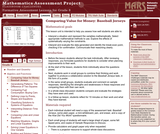Rating

This lesson unit is intended to help teachers assess how well students are able to: interpret a situation and represent the variables mathematically; select appropriate mathematical methods to use; explore the effects of systematically varying the constraints; interpret and evaluate the data generated and identify the break-even point, checking it for confirmation; and communicate their reasoning clearly.

Material Type:
Assessment
Lesson Plan
Provider:
Shell Center for Mathematical Education
U.C. Berkeley
Provider Set:
Mathematics Assessment Project (MAP)
Mathematics Assessment Project (MAP)
04/26/2013Conditions of Use:
No Strings Attached
Rating

This module provides practice problems designed to emphasize basic exponent concepts.

Subject:
Algebra
Material Type:
Homework/Assignment
Provider:
Rice University
Provider Set:
Connexions
Author:
Kenny Felder
02/16/2011Conditions of Use:
No Strings Attached
Rating

This module covers the laws the govern the manipulation of exponents in algebra.

Subject:
Algebra
Material Type:
Provider:
Rice University
Provider Set:
Connexions
Author:
Kenny Felder
02/16/2011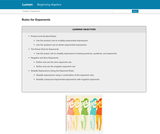Conditions of Use:
Rating

Laws of Exponents

Product and Quotient Rules
The Power rule for Exponents
Negative and Zero Exponents
Simplify Expressions using the Exponent Rules

Subject:
Algebra
Material Type:
Lesson Plan
Provider:
Lumen Learning
12/14/2017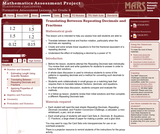Rating

This lesson unit is intended to help teachers assess how well students are able to: translate between decimal and fraction notation, particularly when the decimals are repeating; create and solve simple linear equations to find the fractional equivalent of a repeating decimal; and understand the effect of multiplying a decimal by a power of 10.

Subject:
Algebra
Ratios and Proportions
Material Type:
Assessment
Lesson Plan
Provider:
Shell Center for Mathematical Education
U.C. Berkeley
Provider Set:
Mathematics Assessment Project (MAP)
Mathematics Assessment Project (MAP)
04/26/2013Conditions of Use:
No Strings Attached
Rating

According to the GED testing service, test takers struggle with “applying rules of exponents in numerical expressions with rational exponents to write equivalent expressions with rational exponents.” (https://www.gedtestingservice.com/uploads/files/09738c12fe4e4accd9a16bab7cb99a3c.pdf )
Students do “fairly well” with simple squares and square roots, but there is a “sharp drop-off” when things get more complicated.
These are questions included in the “no calculator” portion of the test.
These skills are Mathematics Standards Level D in the College and Career Readiness Standards for Adult Education (https://www.educateiowa.gov/sites/files/ed/documents/CCRStandardsAdultEd.pdf ) under “Expressions and Equations.”
This curriculum guide will offer opportunities to build the deeper understanding necessary to understand the rules of exponents such as (xm)n = xmn .
Write and evaluate numerical expressions involving whole-number exponents. (6.EE.1)
Know and apply the properties of integer exponents to generate equivalent numerical expressions. (8.EE.1)

Subject:
Mathematics
Material Type:
Activity/Lab
Lesson Plan
Teaching/Learning Strategy
Author:
Susan Jones
05/25/2018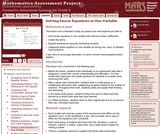Rating

This lesson unit is intended to help teachers assess how well students are able to: solve linear equations in one variable with rational number coefficients; collect like terms; expand expressions using the distributive property; and categorize linear equations in one variable as having one, none, or infinitely many solutions. It also aims to encourage discussion on some common misconceptions about algebra.

Subject:
Algebra
Material Type:
Assessment
Lesson Plan
Provider:
Shell Center for Mathematical Education
U.C. Berkeley
Provider Set:
Mathematics Assessment Project (MAP)
Mathematics Assessment Project (MAP)
04/26/2013Rating

This lesson unit is intended to help teachers assess how well students are able to classify solutions to a pair of linear equations by considering their graphical representations. In particular, this unit aims to help teachers identify and assist students who have difficulties in: using substitution to complete a table of values for a linear equation; identifying a linear equation from a given table of values; and graphing and solving linear equations.

Subject:
Algebra
Material Type:
Assessment
Lesson Plan
Provider:
Shell Center for Mathematical Education
U.C. Berkeley
Provider Set:
Mathematics Assessment Project (MAP)
Mathematics Assessment Project (MAP)
04/26/2013Conditions of Use:
No Strings Attached
Rating

This task requires students to work with very large and small values expressed both in scientific notation and in decimal notation (standard form). In addition, students need to convert units of mass.

Subject:
Mathematics
Material Type:
Activity/Lab
Provider:
Illustrative Mathematics
Provider Set:
Illustrative Mathematics
Author:
Illustrative Mathematics
08/21/2012Rating

This lesson unit is intended to help you assess how well students working with square numbers are able to: choose an appropriate, systematic way to collect and organize data, examining the data for patterns; describe and explain findings clearly and effectively; generalize using numerical, geometrical, graphical and/or algebraic structure; and explain why certain results are possible/impossible, moving towards a proof.

Subject:
Algebra
Geometry
Measurement and Data
Material Type:
Assessment
Lesson Plan
Provider:
Shell Center for Mathematical Education
U.C. Berkeley
Provider Set:
Mathematics Assessment Project (MAP)
Mathematics Assessment Project (MAP)
04/26/2013Rating

This lesson unit is intended to help teachers assess how well students are able to create and solve linear equations. In particular, the lesson will help you identify and help students who have the following difficulties: solving equations with one variable and solving linear equations in more than one way.

Subject:
Algebra
Material Type:
Assessment
Lesson Plan
Provider:
Shell Center for Mathematical Education
U.C. Berkeley
Provider Set:
Mathematics Assessment Project (MAP)
Mathematics Assessment Project (MAP)
04/26/2013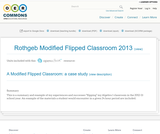Rating

This is a summary and example of my experiences and successes "flipping" my Algebra I classroom in the 2012-13 school year. An example of the materials a student would encounter in a given 24 hour period are included.

Subject:
Mathematics
Material Type:
Case Study
Teaching/Learning Strategy
Author:
Jason Rothgeb
01/28/2016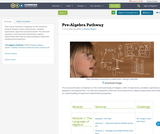Conditions of Use:
Remix and Share
Rating

This course will place a emphasis on the continued study of integers, order of operations, variables, expressions, equations and polynomials. You will solve equations, write and solve proportions, explore polynomials and build an understanding of important mathematical properties.

Subject:
Mathematics
Material Type:
Full Course
Author:
Deanna Mayers
06/10/2018Rating

This lesson unit is intended to help teachers assess how well students are able to: estimate lengths of everyday objects; convert between decimal and scientific notation; and make comparisons of the size of numbers expressed in both decimal and scientific notation.

Material Type:
Assessment
Lesson Plan
Provider:
Shell Center for Mathematical Education
U.C. Berkeley
Provider Set:
Mathematics Assessment Project (MAP)
Mathematics Assessment Project (MAP)
04/26/2013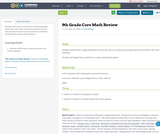Conditions of Use:
Remix and Share
Rating

Students will create an interactive Powerpoint game where they will create real world problems that are used as clues to move on to the next level. Problems include all 8th grade math standards.

Subject:
Mathematics
Material Type:
Interactive
Author:
Linda Ridings
01/28/2016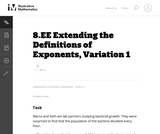Conditions of Use:
No Strings Attached
Rating

This is an instructional task meant to generate a conversation around the meaning of negative integer exponents. While it may be unfamiliar to some students, it is good for them to learn the convention that negative time is simply any time before t=0.

Subject:
Mathematics
Material Type:
Activity/Lab
Provider:
Illustrative Mathematics
Provider Set:
Illustrative Mathematics
Author:
Illustrative Mathematics
05/01/2012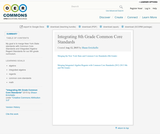Conditions of Use:
No Strings Attached
Rating

My goal is to merge New York State standards with Common Core Standards and Integrated Algebra Regent Standards for our 8th grade curriculum.

Subject:
Mathematics
Material Type:
Full Course
Lesson Plan
Syllabus
Author:
Shaun Errichiello
01/28/2016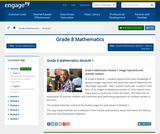Conditions of Use:
Remix and Share
Rating

In Grade 8 Module 1, students expand their basic knowledge of positive integer exponents and prove the Laws of Exponents for any integer exponent.  Next, students work with numbers in the form of an integer multiplied by a power of 10 to express how many times as much one is than the other.  This leads into an explanation of scientific notation and continued work performing operations on numbers written in this form.

Subject:
Numbers and Operations
Material Type:
Module
Provider:
New York State Education Department
Provider Set:
EngageNY